# NCERT Exemplar Class 12 Chemistry Solutions for Chapter 4 - Chemical Kinetics

The NCERT Class 12 Chemistry Exemplar for Chapter 4 Chemical Kinetics is an important study material for students who are preparing for the CBSE Class 12 Board examination and entrance exams like NEET and JEE. The NCERT Class 12 Chemistry Exemplar for Chapter 4 PDF has exemplar questions taken from the NCERT Exemplar Class 12 Chemistry book, along with chemical kinetics exemplar, prepared by chemistry subject experts of BYJU’S, and chemical kinetics problems for Class 12. These exemplar solutions have a variety of questions like MCQs, fill in the blanks, match the following, numerical problems, exercises and assignments that will help you to grasp the concepts involved in the chapter Chemical Kinetics.

## Download the PDF of NCERT Exemplar Solutions for Class 12 Chemistry Chapter 4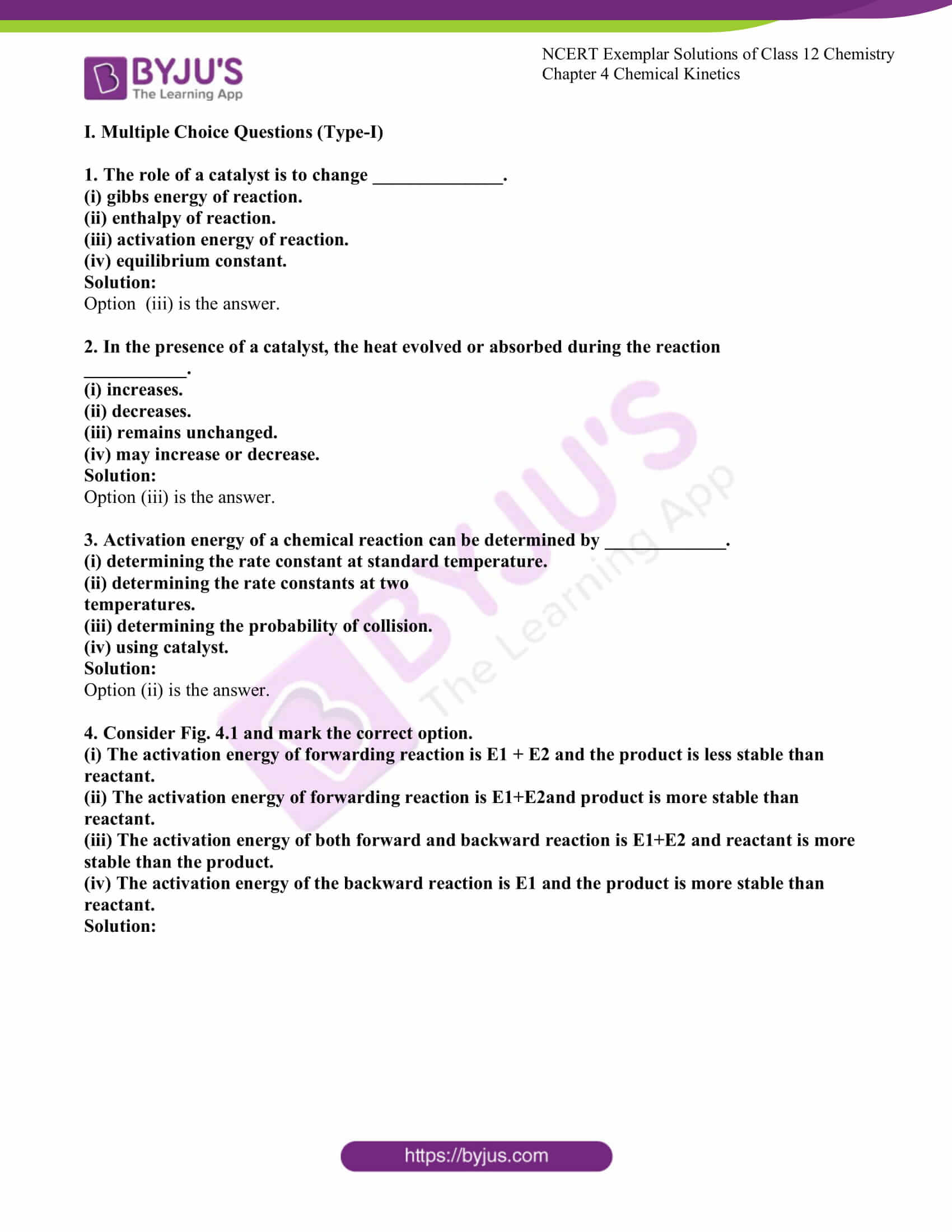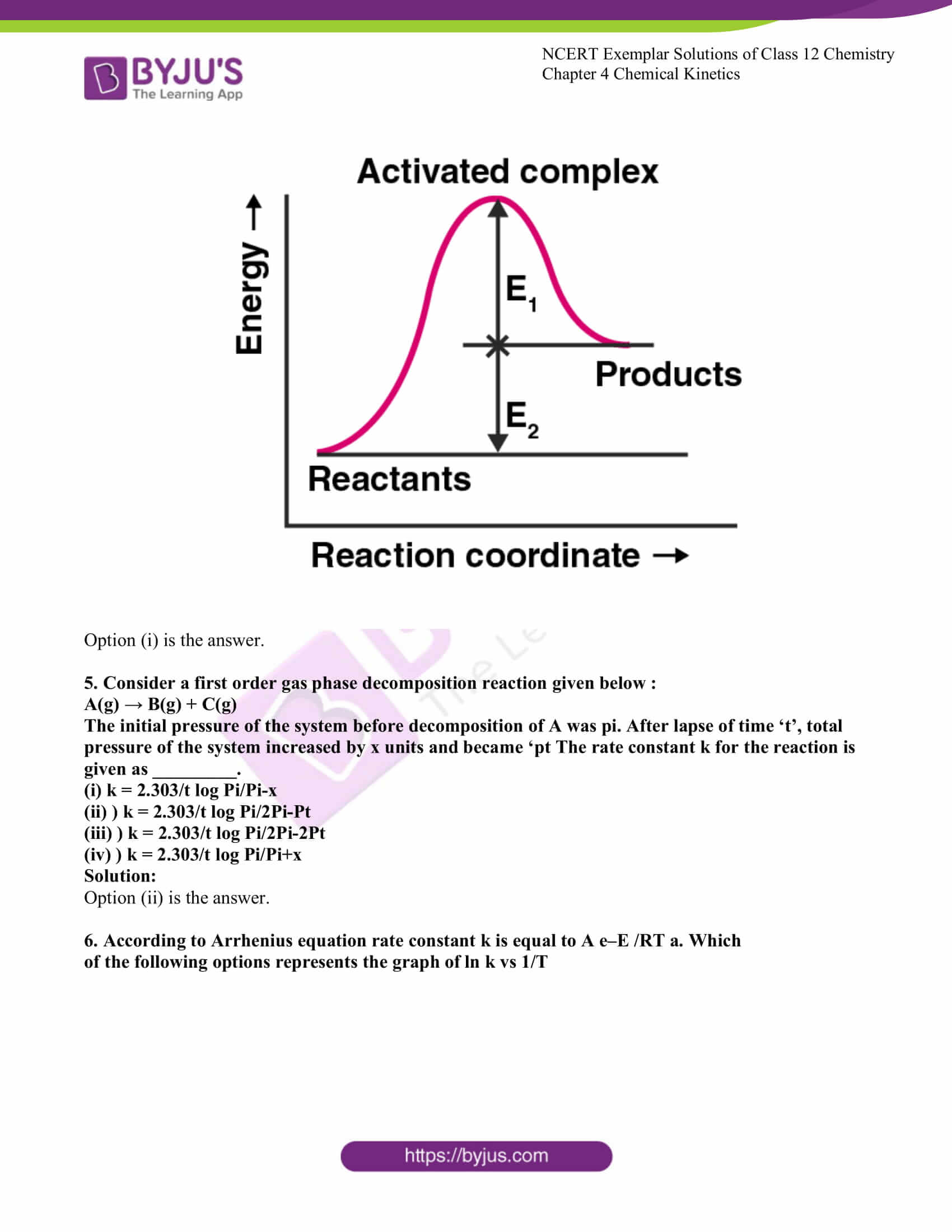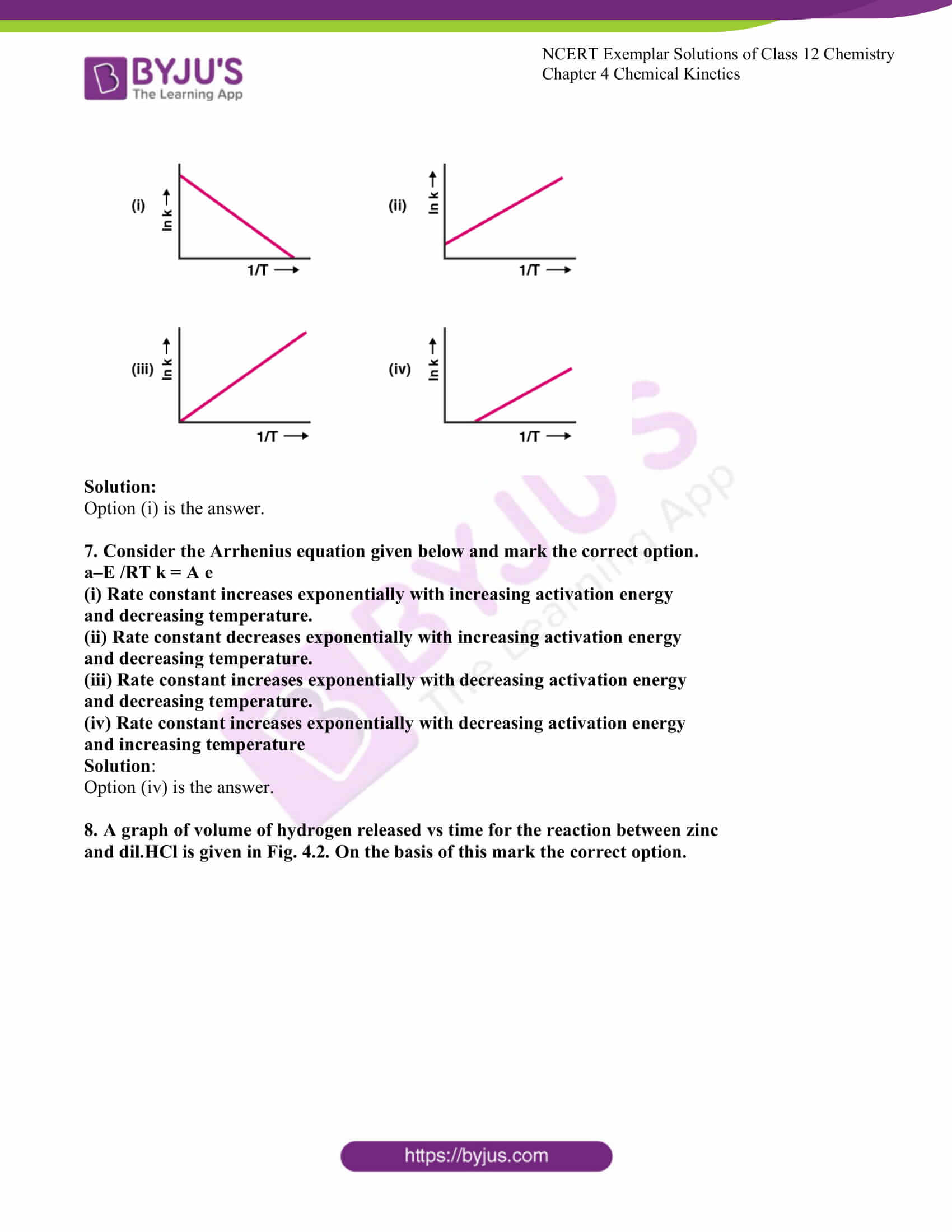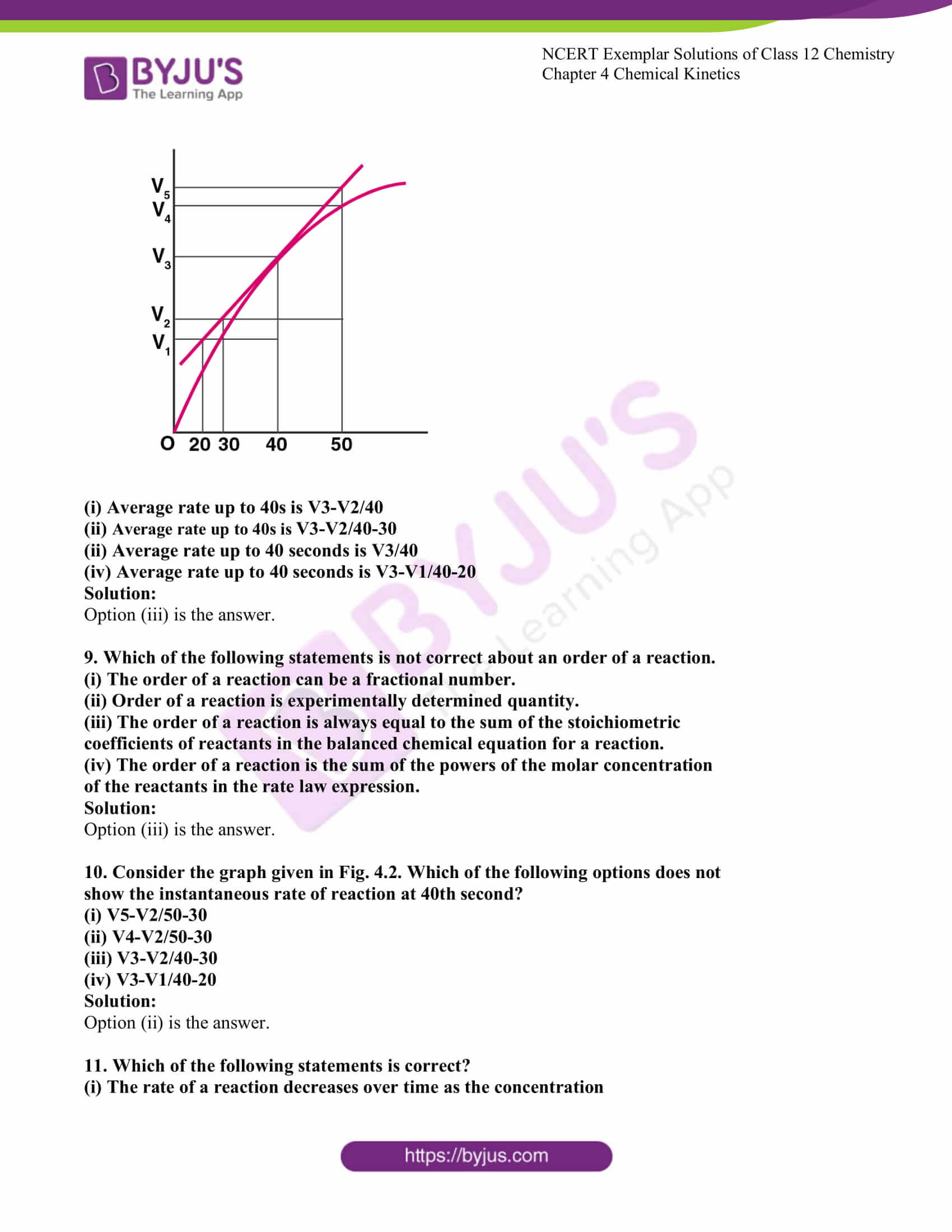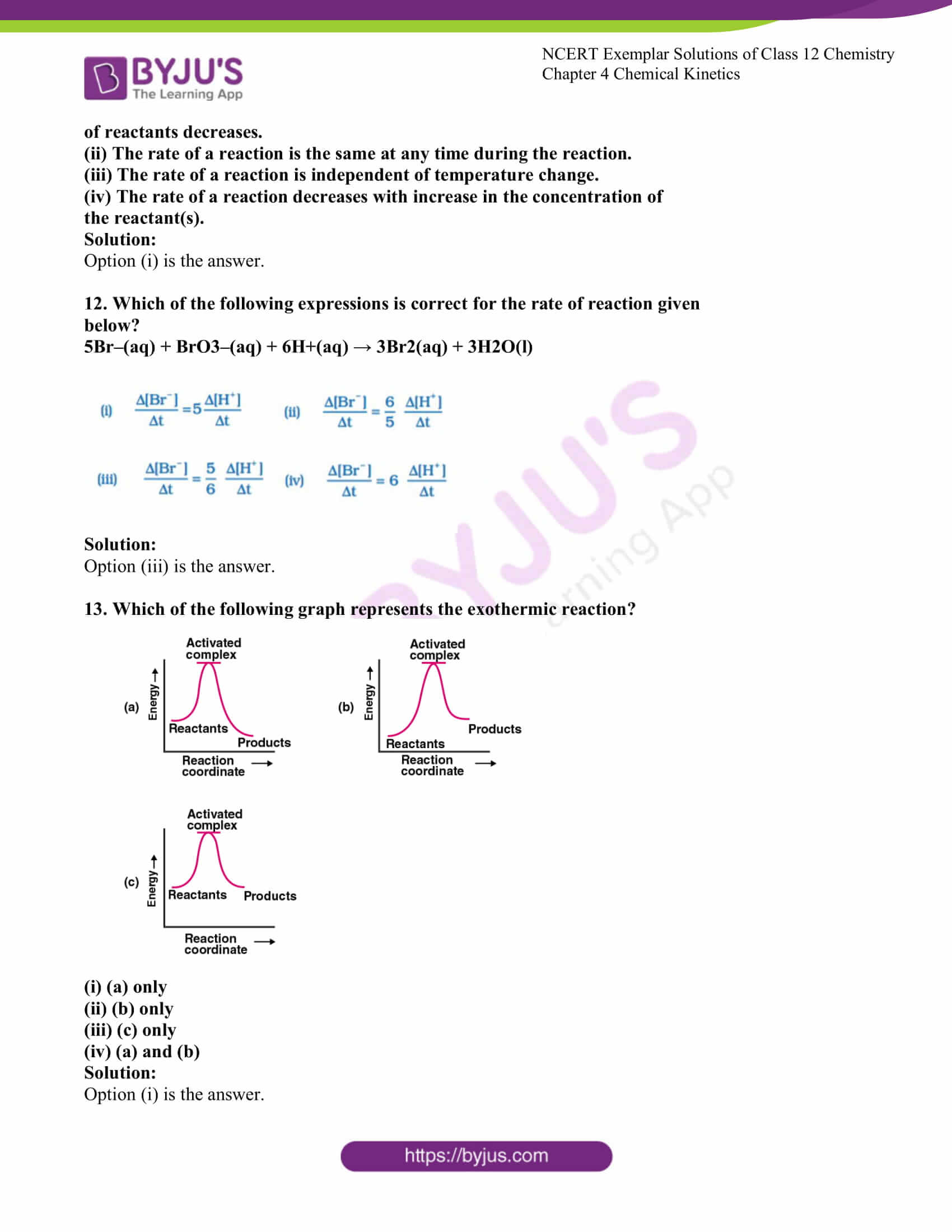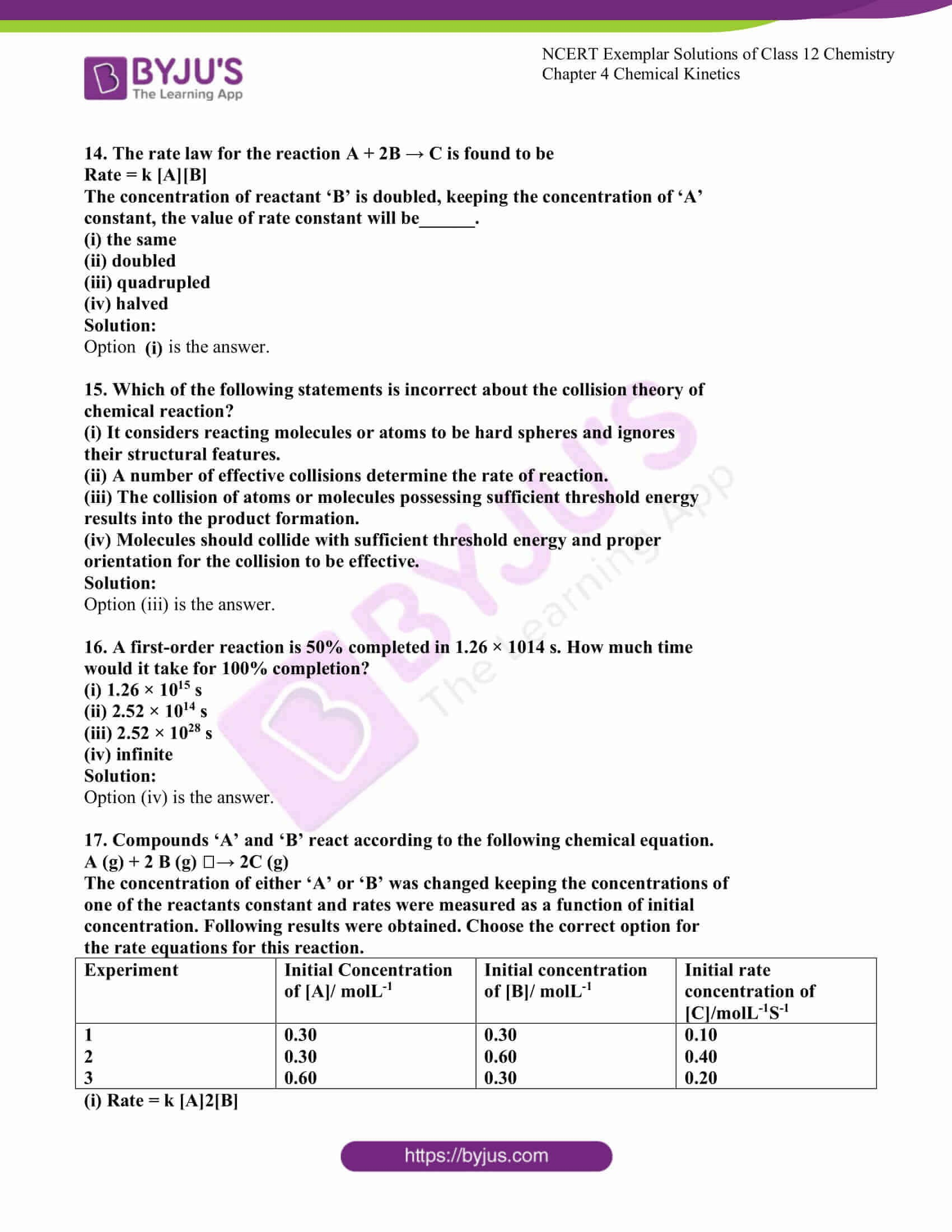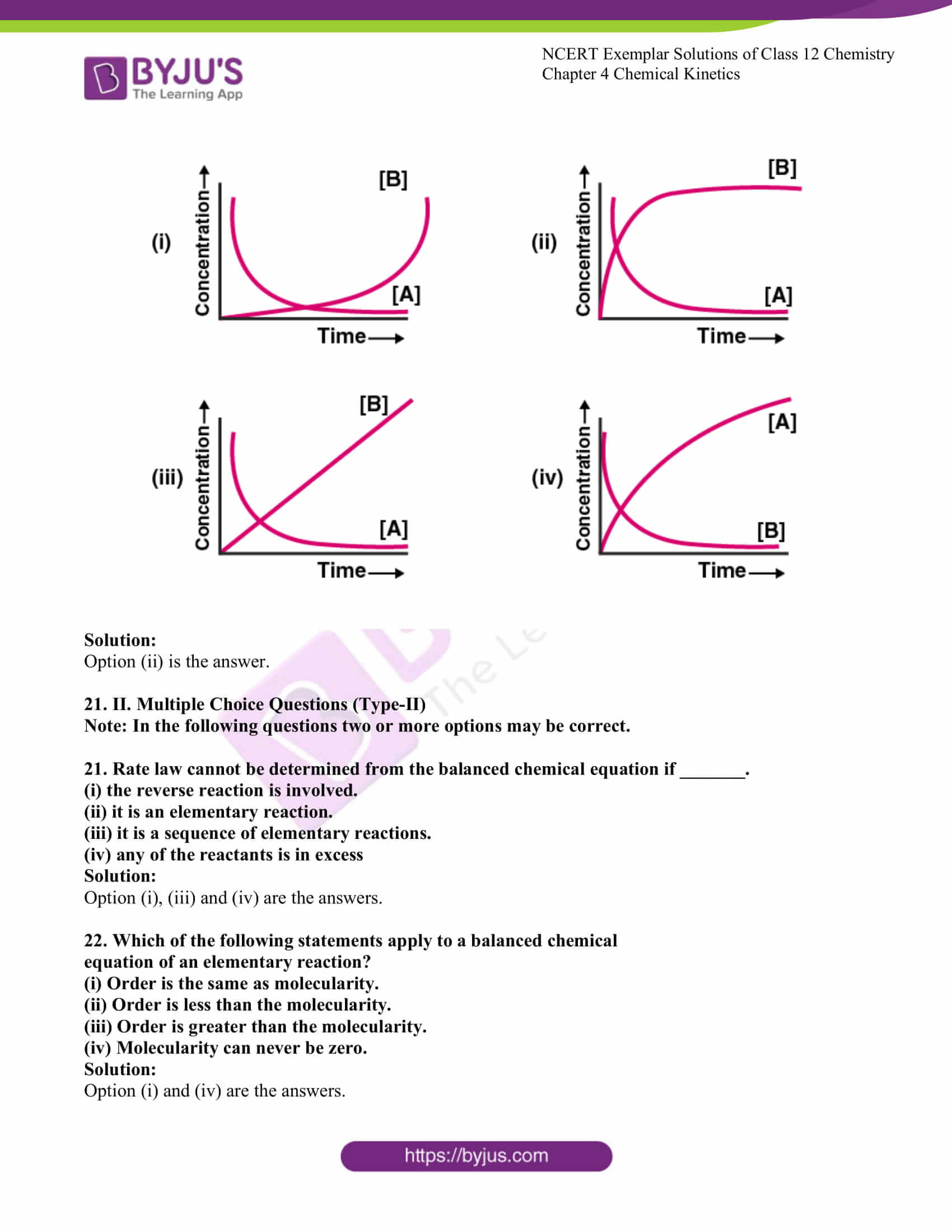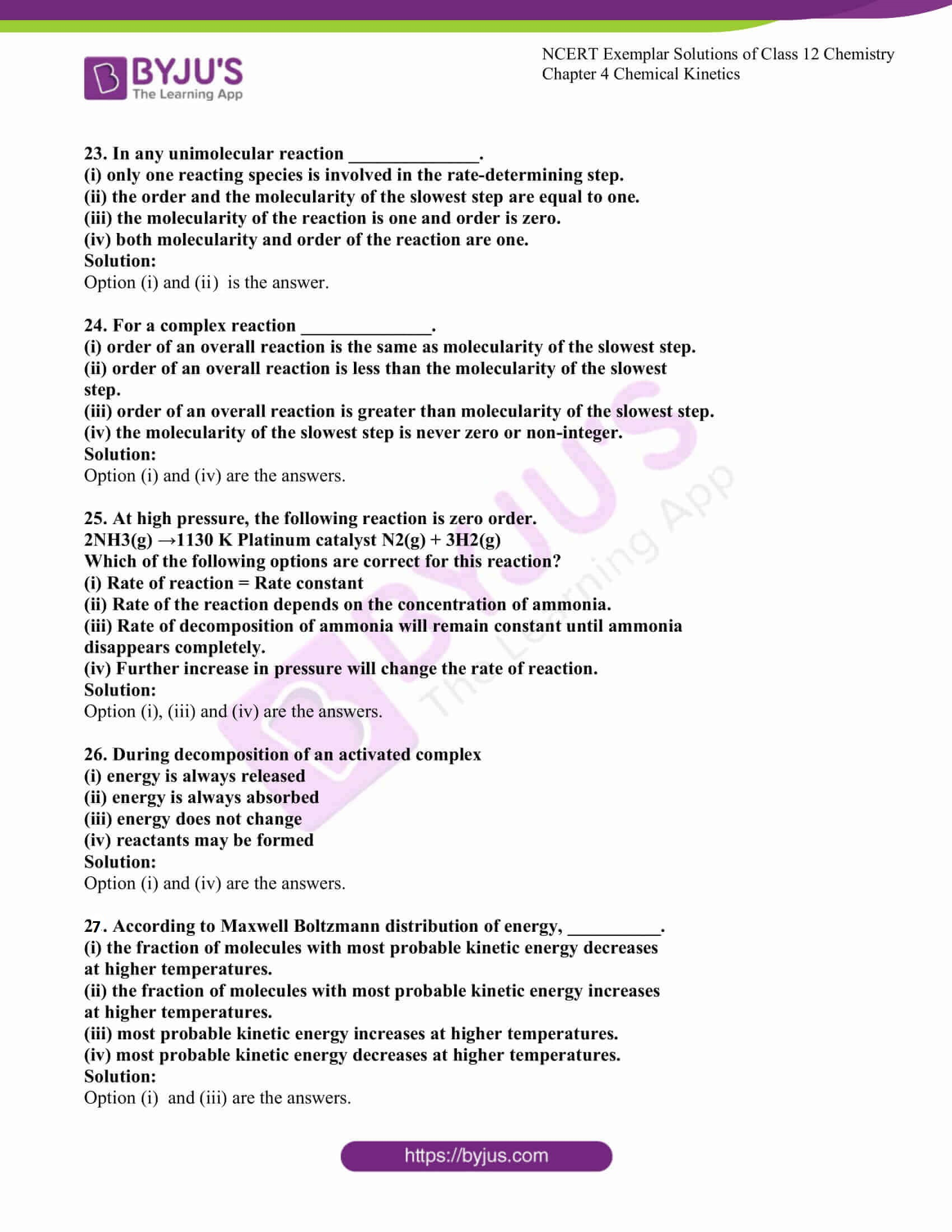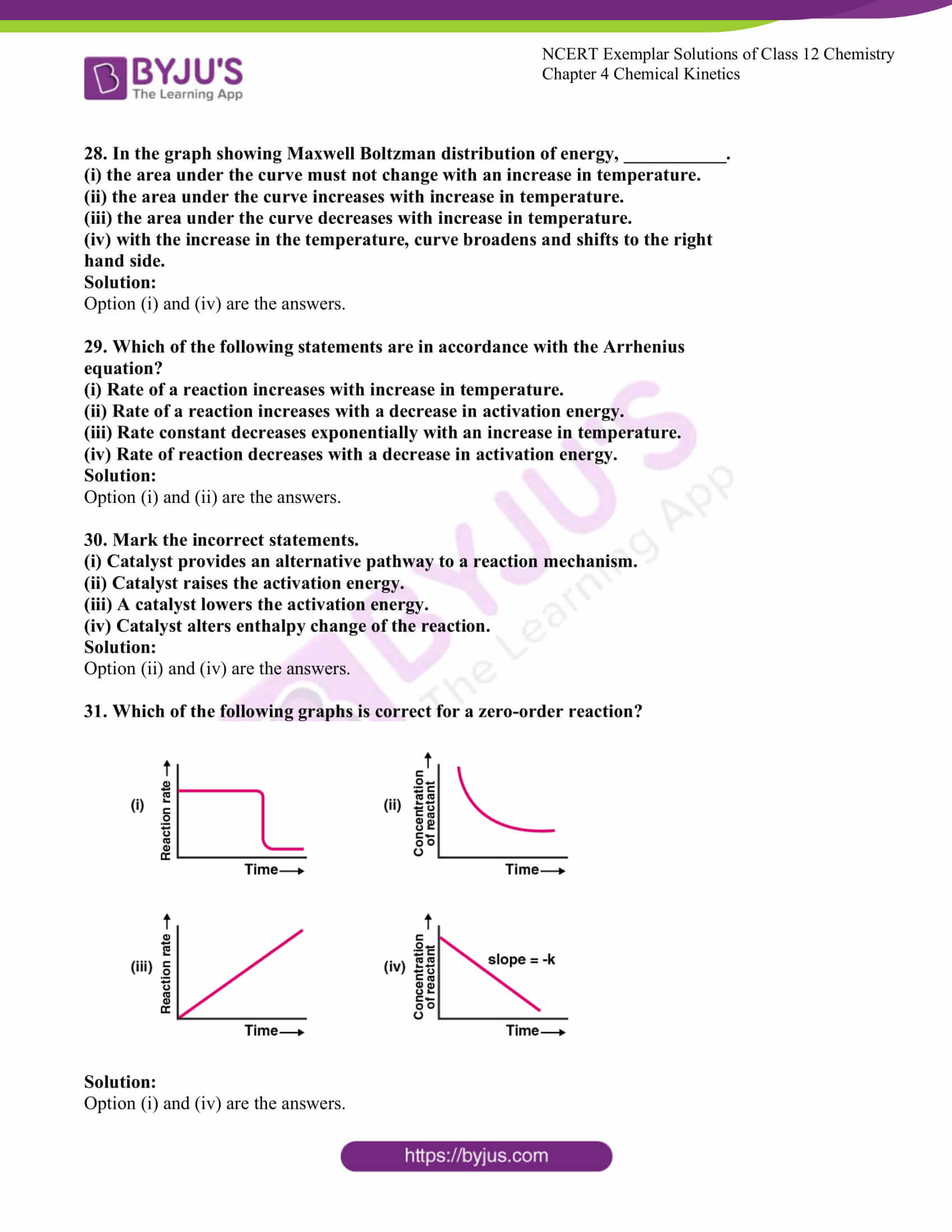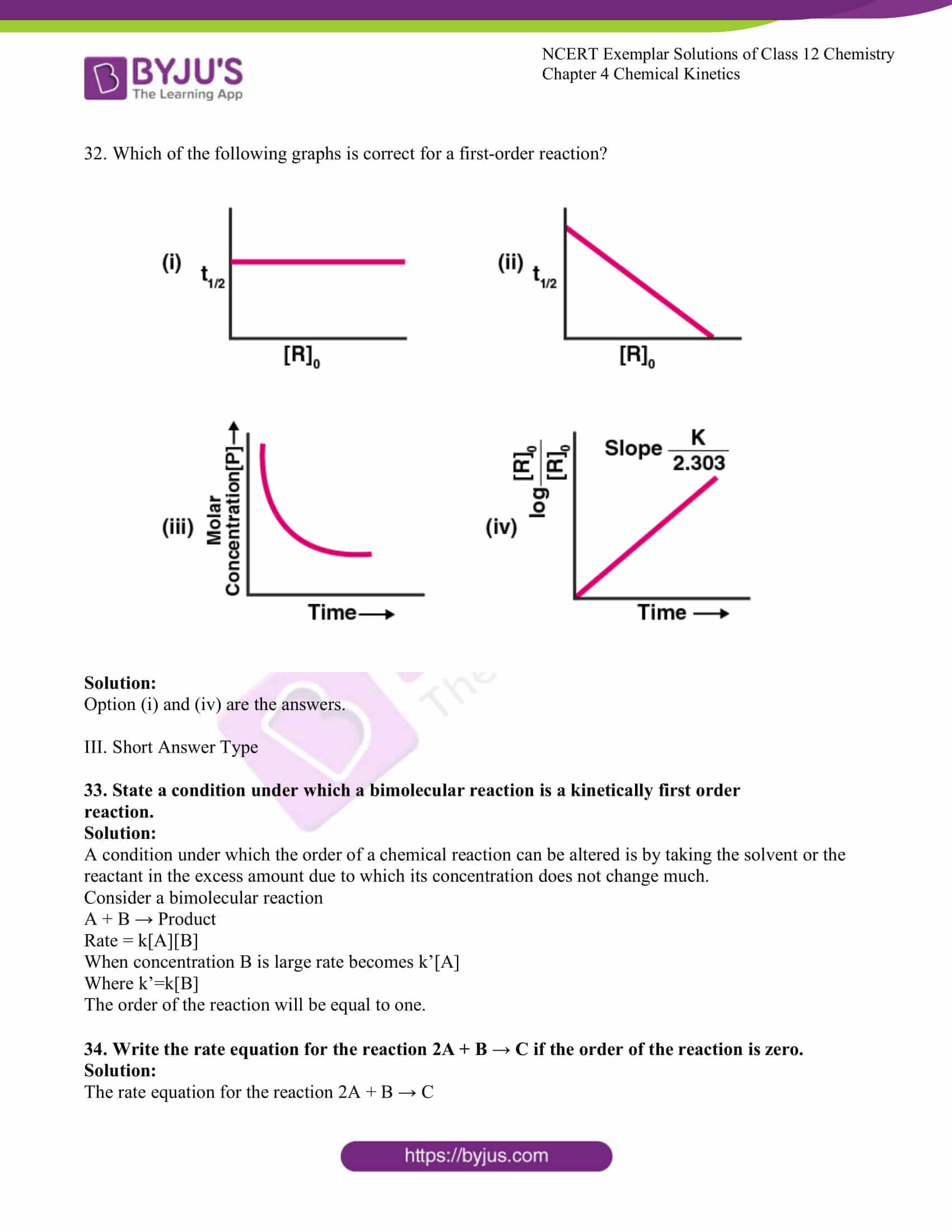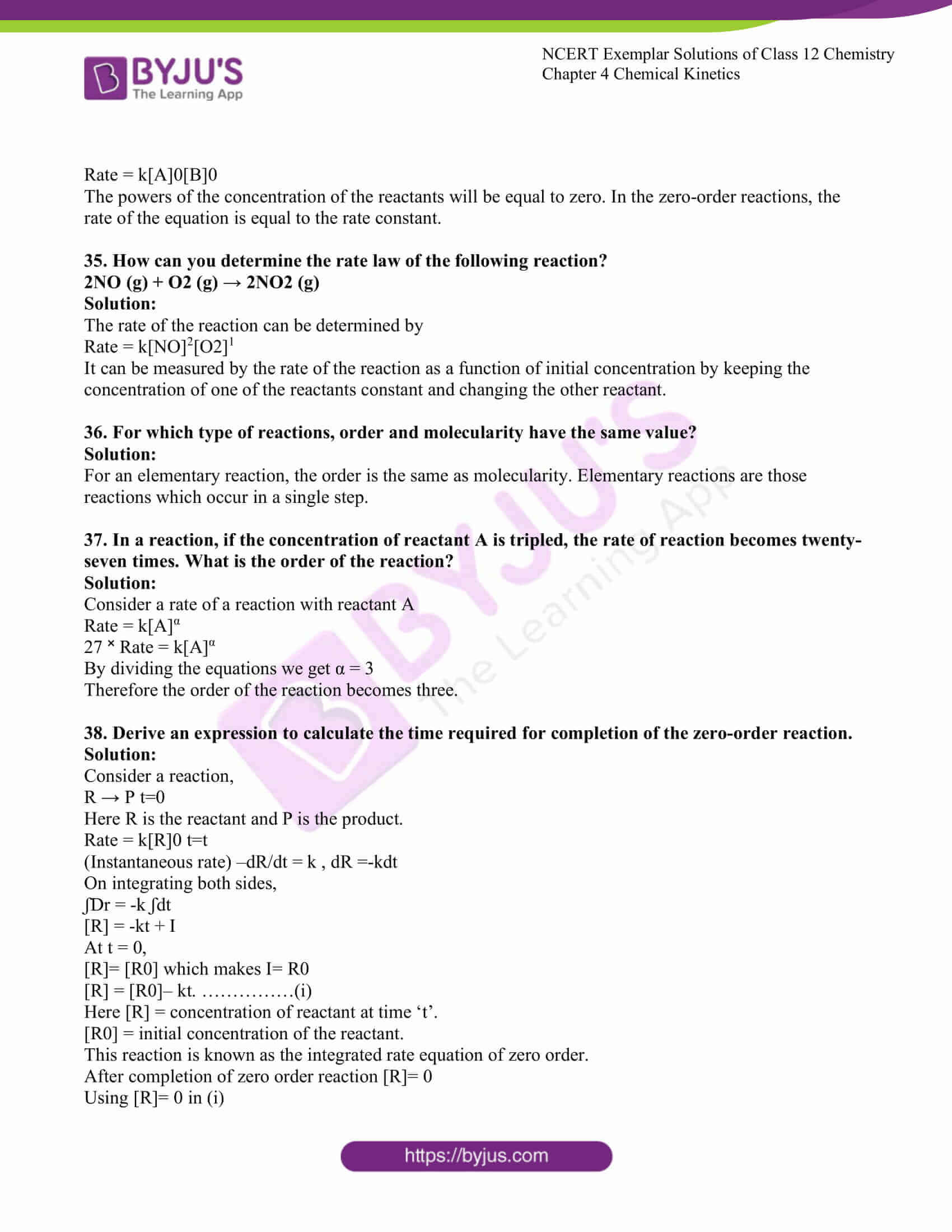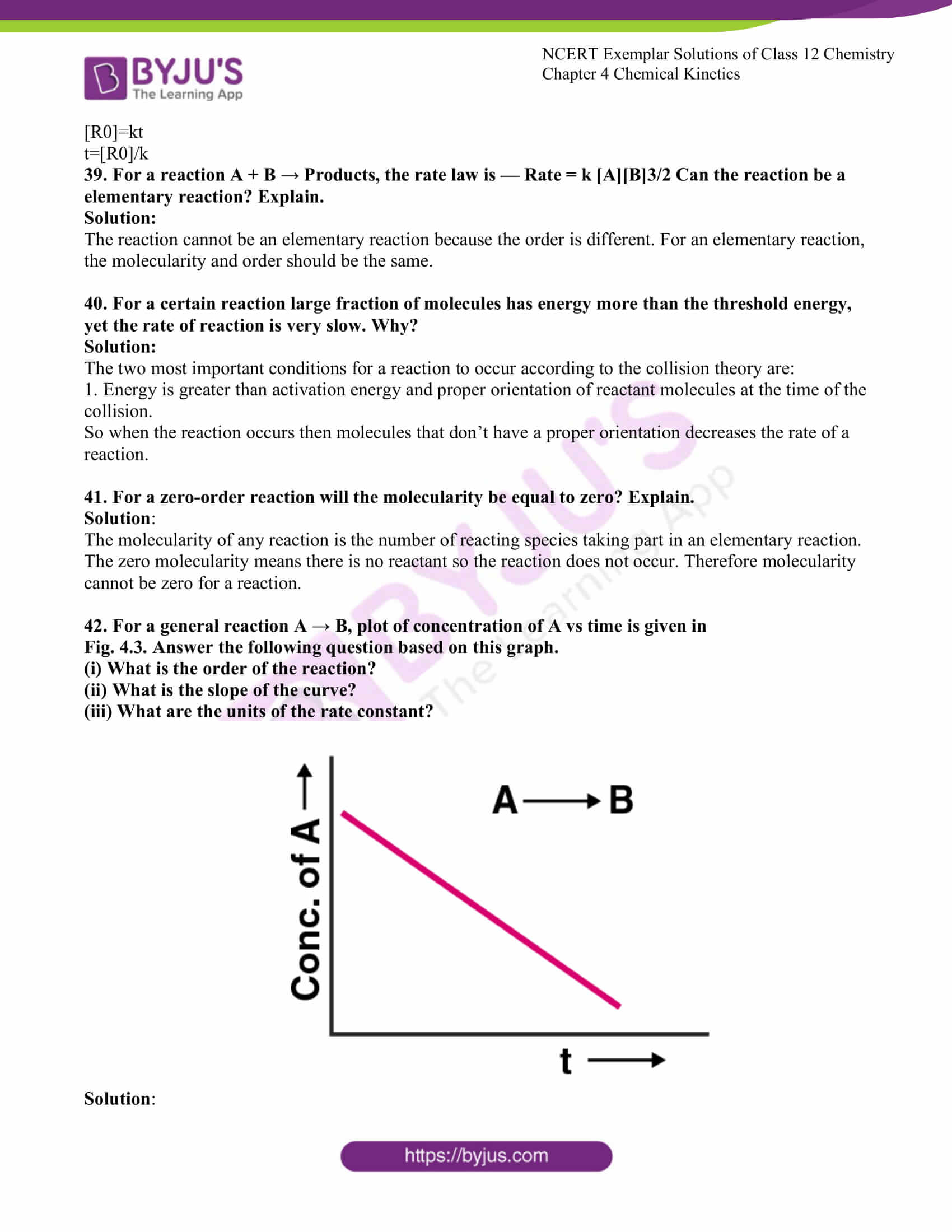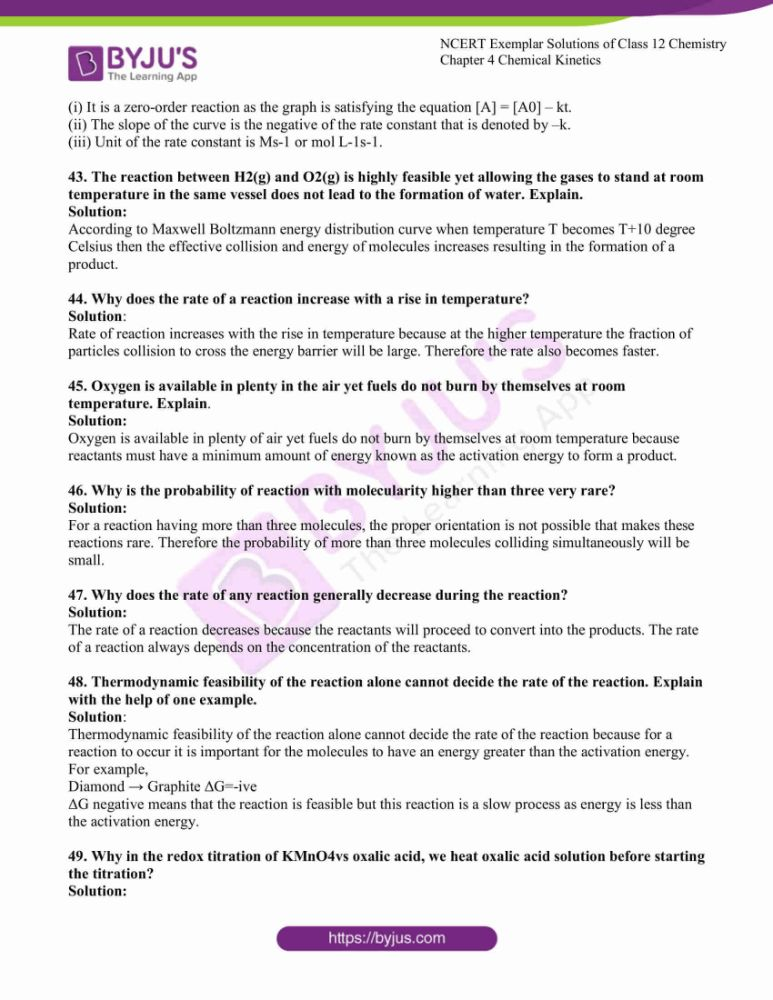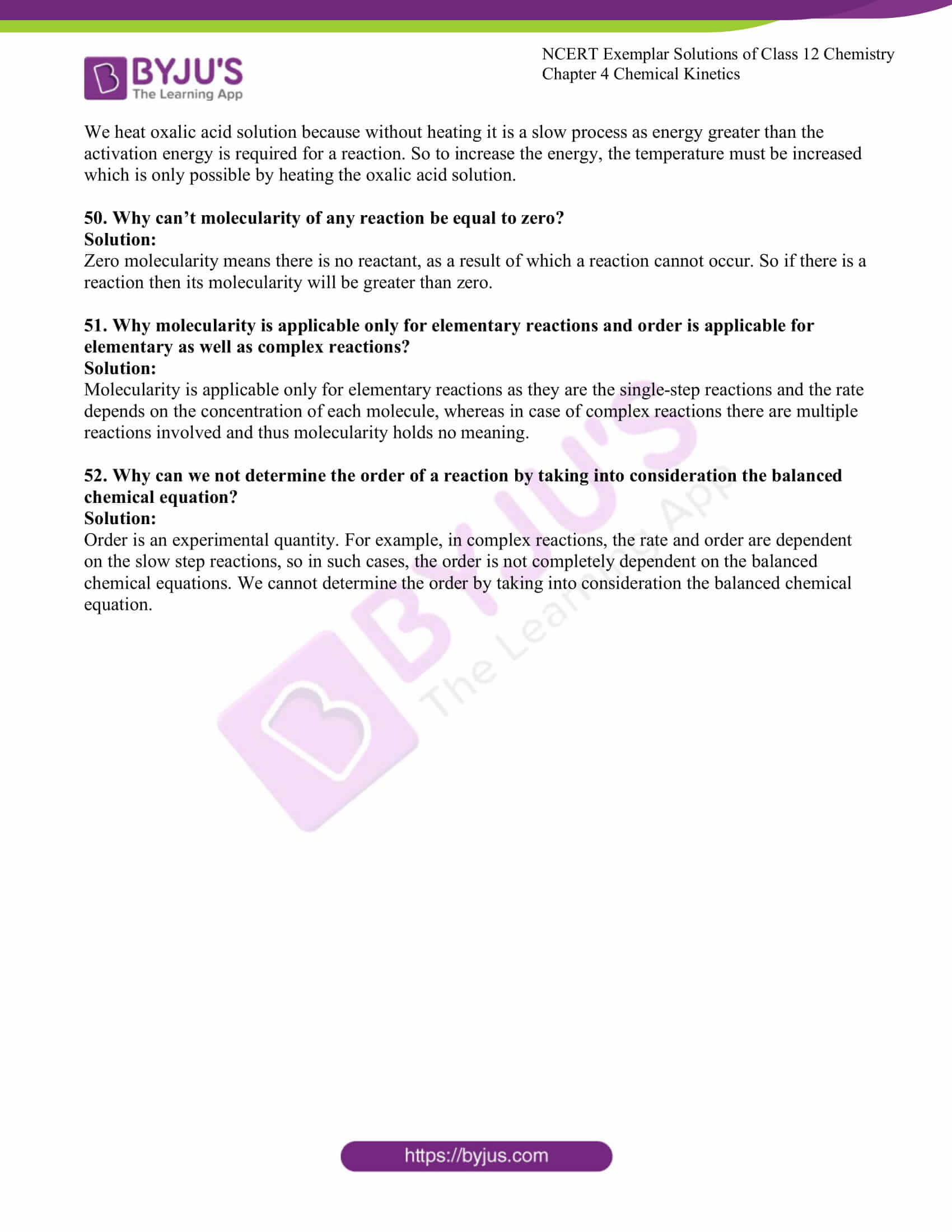### Access Solutions to the NCERT Exemplar Class 12 Chemistry Chapter 4

I. Multiple Choice Questions (Type-I)

1. The role of a catalyst is to change ______________.

(i) gibbs energy of reaction.

(ii) enthalpy of reaction.

(iii) activation energy of reaction.

(iv) equilibrium constant.

Solution:

2. In the presence of a catalyst, the heat evolved or absorbed during the reaction

___________.

(i) increases.

(ii) decreases.

(iii) remains unchanged.

(iv) may increase or decrease.

Solution:

3. Activation energy of a chemical reaction can be determined by _____________.

(i) determining the rate constant at standard temperature.

(ii) determining the rate constants at two

temperatures.

(iii) determining the probability of collision.

(iv) using catalyst.

Solution:

4. Consider Fig. 4.1 and mark the correct option.

(i) The activation energy of forwarding reaction is E1 + E2 and the product is less stable than reactant.

(ii) The activation energy of forwarding reaction is E1+E2and product is more stable than reactant.

(iii) The activation energy of both forward and backward reaction is E1+E2 and reactant is more stable than the product.

(iv) The activation energy of the backward reaction is E1 and the product is more stable than reactant.

Solution: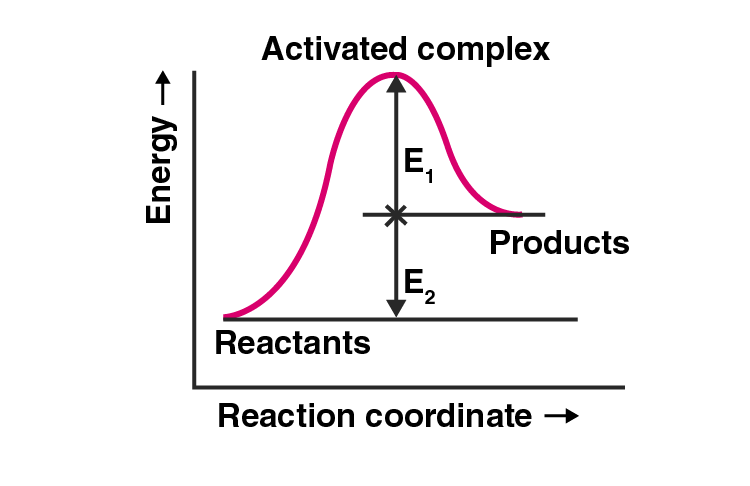5. Consider a first order gas phase decomposition reaction given below :

A(g) → B(g) + C(g)

The initial pressure of the system before decomposition of A was pi. After lapse of time ‘t’, total pressure of the system increased by x units and became ‘pt The rate constant k for the reaction is given as _________.

(i) k = 2.303/t log Pi/Pi-x

(ii) ) k = 2.303/t log Pi/2Pi-Pt

(iii) ) k = 2.303/t log Pi/2Pi-2Pt

(iv) ) k = 2.303/t log Pi/Pi+x

Solution:

6. According to Arrhenius equation rate constant k is equal to A e–E /RT a. Which

of the following options represents the graph of ln k vs 1/T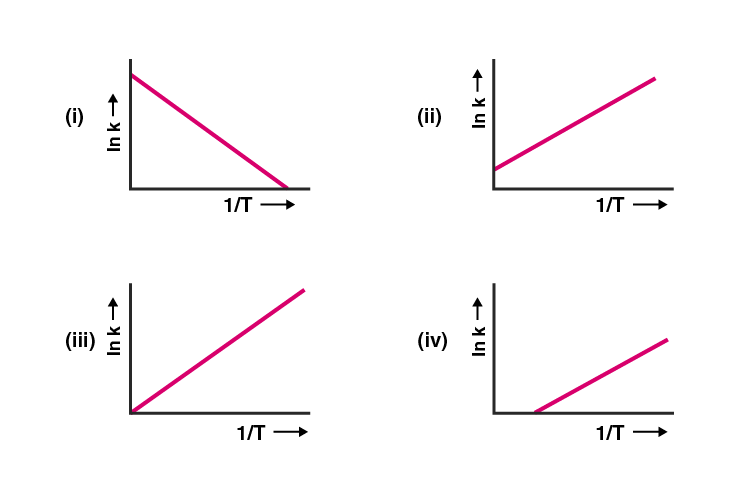Solution:

7. Consider the Arrhenius equation given below and mark the correct option.

a–E /RT k = A e

(i) Rate constant increases exponentially with increasing activation energy

and decreasing temperature.

(ii) Rate constant decreases exponentially with increasing activation energy

and decreasing temperature.

(iii) Rate constant increases exponentially with decreasing activation energy

and decreasing temperature.

(iv) Rate constant increases exponentially with decreasing activation energy

and increasing temperature

Solution:

8. A graph of volume of hydrogen released vs time for the reaction between zinc

and dil.HCl is given in Fig. 4.2. On the basis of this mark the correct option.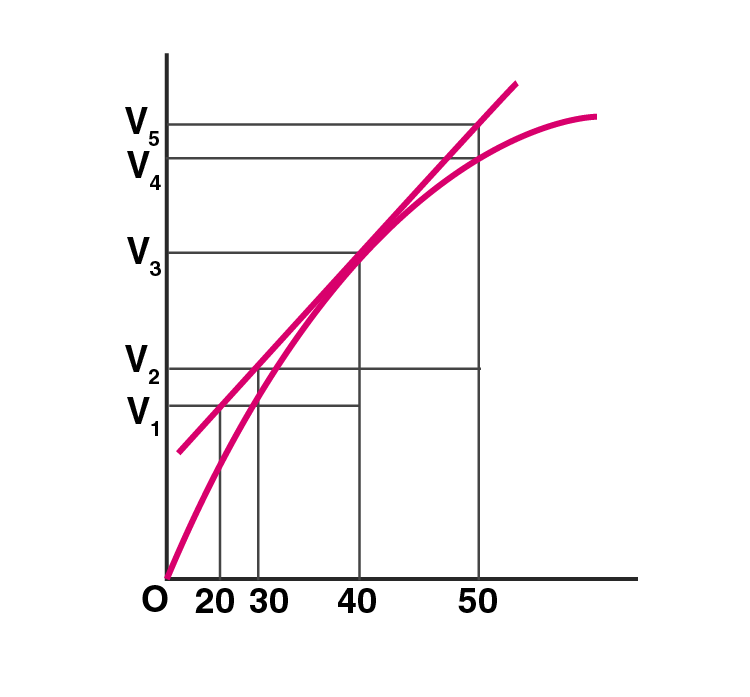(i) Average rate up to 40s is V3-V2/40

(ii) Average rate up to 40s is V3-V2/40-30

(ii) Average rate up to 40 seconds is V3/40

(iv) Average rate up to 40 seconds is V3-V1/40-20

Solution:

9. Which of the following statements is not correct about an order of a reaction.

(i) The order of a reaction can be a fractional number.

(ii) Order of a reaction is experimentally determined quantity.

(iii) The order of a reaction is always equal to the sum of the stoichiometric

coefficients of reactants in the balanced chemical equation for a reaction.

(iv) The order of a reaction is the sum of the powers of the molar concentration

of the reactants in the rate law expression.

Solution:

10. Consider the graph given in Fig. 4.2. Which of the following options does not

show the instantaneous rate of reaction at 40th second?

(i) V5-V2/50-30

(ii) V4-V2/50-30

(iii) V3-V2/40-30

(iv) V3-V1/40-20

Solution:

11. Which of the following statements is correct?

(i) The rate of a reaction decreases over time as the concentration

of reactants decreases.

(ii) The rate of a reaction is the same at any time during the reaction.

(iii) The rate of a reaction is independent of temperature change.

(iv) The rate of a reaction decreases with increase in the concentration of

the reactant(s).Solution:

12. Which of the following expressions is correct for the rate of reaction given

below?

5Br–(aq) + BrO3–(aq) + 6H+(aq) → 3Br2(aq) + 3H2O(l)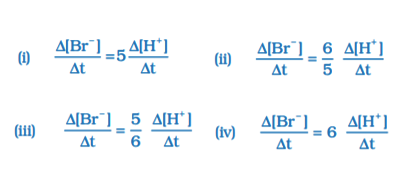Solution:

13. Which of the following graph represents the exothermic reaction?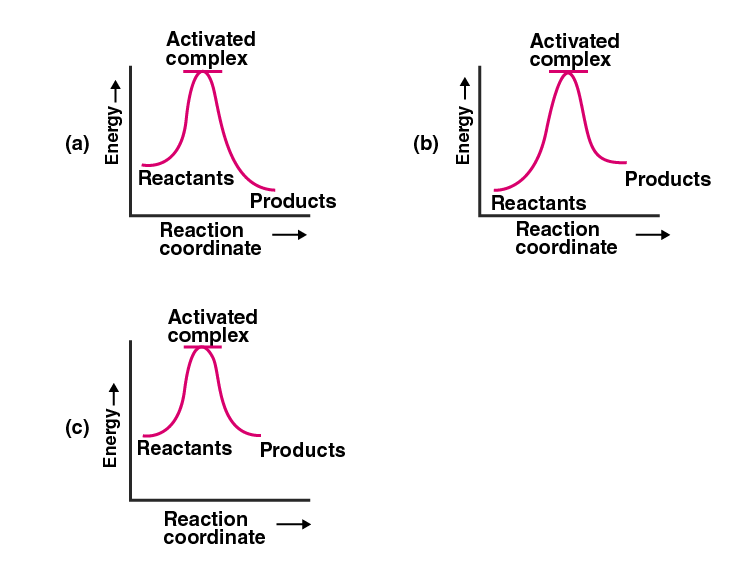(i) (a) only

(ii) (b) only

(iii) (c) only

(iv) (a) and (b)

Solution:

14. The rate law for the reaction A + 2B → C is found to be

Rate = k [A][B]

The concentration of reactant ‘B’ is doubled, keeping the concentration of ‘A’

constant, the value of rate constant will be______.

(i) the same

(ii) doubled

(iv) halved

Solution:

15. Which of the following statements is incorrect about the collision theory of

chemical reaction?

(i) It considers reacting molecules or atoms to be hard spheres and ignores

their structural features.

(ii) A number of effective collisions determine the rate of reaction.

(iii) The collision of atoms or molecules possessing sufficient threshold energy

results into the product formation.

(iv) Molecules should collide with sufficient threshold energy and proper

orientation for the collision to be effective.

Solution:

16. A first-order reaction is 50% completed in 1.26 × 1014 s. How much time

would it take for 100% completion?

(i) 1.26 × 1015 s

(ii) 2.52 × 1014 s

(iii) 2.52 × 1028 s

(iv) infinite

Solution:

17. Compounds ‘A’ and ‘B’ react according to the following chemical equation.

A (g) + 2 B (g) → 2C (g)

The concentration of either ‘A’ or ‘B’ was changed keeping the concentrations of

one of the reactants constant and rates were measured as a function of initial

concentration. Following results were obtained. Choose the correct option for

the rate equations for this reaction.

 Experiment Initial Concentration of [A]/ molL-1 Initial concentration of [B]/ molL-1 Initial rate concentration of [C]/molL-1S-1 1 2 3 0.30 0.30 0.60 0.30 0.60 0.30 0.10 0.40 0.20

(i) Rate = k [A]2[B]

(ii) Rate = k [A] [B]2

(iii) Rate = k [A] [B]

(iv) Rate = k [A]2[B]0

Solution:

18. Which of the following statement is not correct for the catalyst?

(i) It catalyses the forward and backward reaction to the same extent.

(ii) It alters ∆G of the reaction.

(iii) It is a substance that does not change the equilibrium constant of a

reaction.

(iv) It provides an alternate mechanism by reducing activation energy

between reactants and products.

Solution:

19. The value of rate constant of a pseudo first order reaction ____________.

(i) depends on the concentration of reactants present in a small amount.

(ii) depends on the concentration of reactants present in excess.

(iii) is independent of the concentration of reactants.

(iv) depends only on temperature.Solution:

20. Consider the reaction A B. The concentration of both the reactants and

the products varies exponentially with time. Which of the following figures

correctly describes the change in concentration of reactants and products

with time?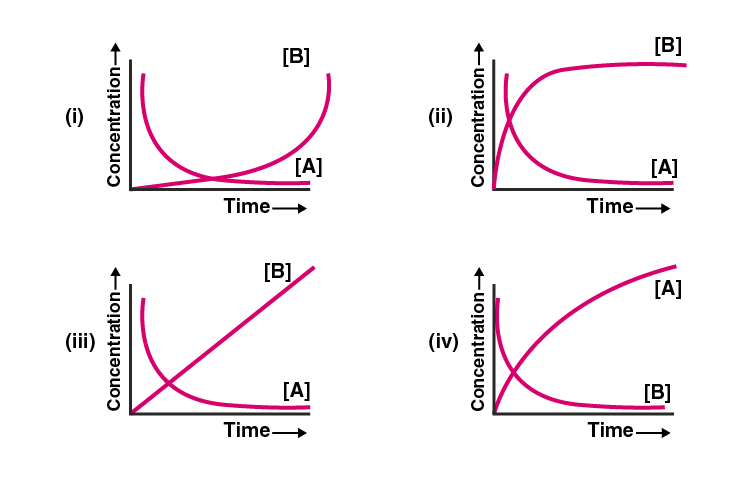Solution:

21. II. Multiple Choice Questions (Type-II)

Note: In the following questions two or more options may be correct.

21. Rate law cannot be determined from the balanced chemical equation if _______.

(i) the reverse reaction is involved.

(ii) it is an elementary reaction.

(iii) it is a sequence of elementary reactions.

(iv) any of the reactants is in excess

Solution:

Option (i), (iii) and (iv) are the answers.

22. Which of the following statements apply to a balanced chemical

equation of an elementary reaction?

(i) Order is the same as molecularity.

(ii) Order is less than the molecularity.

(iii) Order is greater than the molecularity.

(iv) Molecularity can never be zero.

Solution:

Option (i) and (iv) are the answers.

23. In any unimolecular reaction ______________.

(i) only one reacting species is involved in the rate-determining step.

(ii) the order and the molecularity of the slowest step are equal to one.

(iii) the molecularity of the reaction is one and order is zero.

(iv) both molecularity and order of the reaction are one.

Solution:

Option (i) and (ii) is the answer.

24. For a complex reaction ______________.

(i) order of an overall reaction is the same as molecularity of the slowest step.

(ii) order of an overall reaction is less than the molecularity of the slowest

step.

(iii) order of an overall reaction is greater than molecularity of the slowest step.

(iv) the molecularity of the slowest step is never zero or non-integer.

Solution:

Option (i) and (iv) are the answers.

25. At high pressure, the following reaction is zero order.

2NH3(g) →1130 K Platinum catalyst N2(g) + 3H2(g)

Which of the following options are correct for this reaction?

(i) Rate of reaction = Rate constant

(ii) Rate of the reaction depends on the concentration of ammonia.

(iii) Rate of decomposition of ammonia will remain constant until ammonia

disappears completely.

(iv) Further increase in pressure will change the rate of reaction.

Solution:

Option (i), (iii) and (iv) are the answers.

26. During decomposition of an activated complex

(i) energy is always released

(ii) energy is always absorbed

(iii) energy does not change

(iv) reactants may be formed

Solution:

Option (i) and (iv) are the answers.

27. According to Maxwell Boltzmann distribution of energy, __________.

(i) the fraction of molecules with most probable kinetic energy decreases

at higher temperatures.

(ii) the fraction of molecules with most probable kinetic energy increases

at higher temperatures.

(iii) most probable kinetic energy increases at higher temperatures.

(iv) most probable kinetic energy decreases at higher temperatures.

Solution:

Option (i) and (iii) are the answers.

28. In the graph showing Maxwell Boltzman distribution of energy, ___________.

(i) the area under the curve must not change with an increase in temperature.

(ii) the area under the curve increases with increase in temperature.

(iii) the area under the curve decreases with increase in temperature.

(iv) with the increase in the temperature, curve broadens and shifts to the right-hand side.

Solution:

Option (i) and (iv) are the answers.

29. Which of the following statements are in accordance with the Arrhenius

equation?

(i) Rate of a reaction increases with increase in temperature.

(ii) Rate of a reaction increases with a decrease in activation energy.

(iii) Rate constant decreases exponentially with an increase in temperature.

(iv) Rate of reaction decreases with a decrease in activation energy.Solution:

Option (i) and (ii) are the answers.

30. Mark the incorrect statements.

(i) Catalyst provides an alternative pathway to a reaction mechanism.

(ii) Catalyst raises the activation energy.

(iii) A catalyst lowers the activation energy.

(iv) Catalyst alters enthalpy change of the reaction.

Solution:

Option (ii) and (iv) are the answers.

31. Which of the following graphs is correct for a zero-order reaction?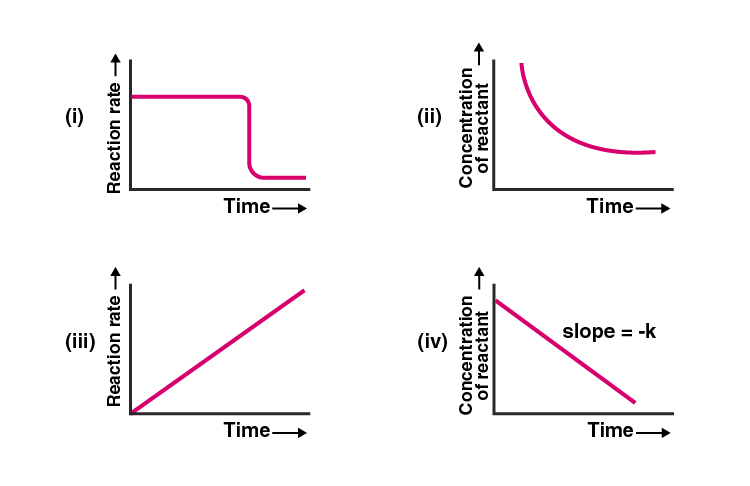Solution:

Option (ii) and (iv) are the answers.

32. Which of the following graphs is correct for a first-order reaction?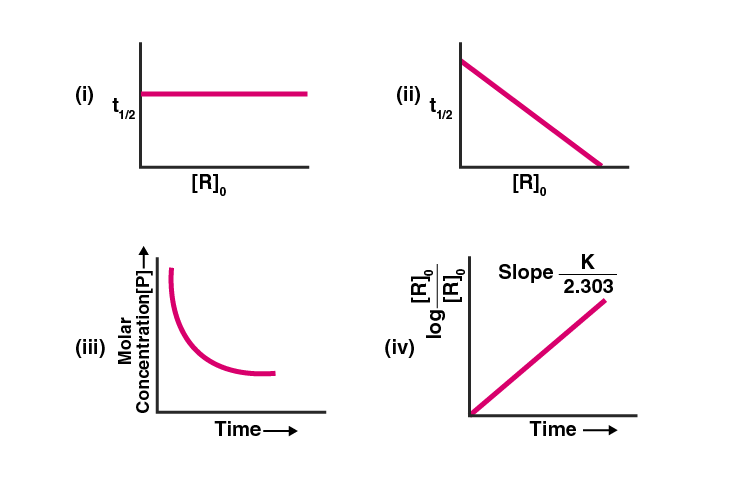Solution:

Option (i) and (iv) are the answers.

33. State a condition under which a bimolecular reaction is a kinetically first-order

reaction.

Solution:

A condition under which the order of a chemical reaction can be altered is by taking the solvent or the reactant in the excess amount due to which its concentration does not change much.

Consider a bimolecular reaction

A + B → Product

Rate = k[A][B]

When concentration B is large rate becomes k’[A]

Where k’=k[B]

The order of the reaction will be equal to one.

34. Write the rate equation for the reaction 2A + B → C if the order of the reaction is zero.

Solution:

The rate equation for the reaction 2A + B → C

Rate = k[A]0[B]0

The powers of the concentration of the reactants will be equal to zero. In the zero-order reactions, the rate of the equation is equal to the rate constant.

35. How can you determine the rate law of the following reaction?

2NO (g) + O2 (g) → 2NO2 (g)

Solution:

The rate of the reaction can be determined by

Rate = k[NO]2[O2]1

It can be measured by the rate of the reaction as a function of initial concentration by keeping the concentration of one of the reactants constant and changing the other reactant.

36. For which type of reactions, order and molecularity have the same value?

Solution:

For an elementary reaction, the order is the same as molecularity. Elementary reactions are those reactions which occur in a single step.

37. In a reaction, if the concentration of reactant A is tripled, the rate of reaction becomes twenty-seven times. What is the order of the reaction?

Solution:

Consider a rate of a reaction with reactant A

Rate = k[A]α

27 ˟ Rate = k[A]α

By dividing the equations we get α = 3

Therefore the order of the reaction becomes three.

38. Derive an expression to calculate the time required for completion of the zero-order reaction.

Solution:

Consider a reaction,

R → P t=0

Here R is the reactant and P is the product.

Rate = k[R]0 t=t

(Instantaneous rate) –dR/dt = k , dR =-kdt

On integrating both sides,

ʃDr = -k ʃdt

[R] = -kt + I

At t = 0,

[R]= [R0] which makes I= R0

[R] = [R0]– kt. ……………(i)

Here [R] = concentration of reactant at time ‘t’.

[R0] = initial concentration of the reactant.

This reaction is known as the integrated rate equation of zero order.

After completion of zero order reaction [R]= 0

Using [R]= 0 in (i)

[R0]=kt

t=[R0]/k

39. For a reaction A + B → Products, the rate law is — Rate = k [A][B]3/2 Can the reaction be an elementary reaction? Explain.

Solution:

The reaction cannot be an elementary reaction because the order is different. For an elementary reaction, the molecularity and order should be the same.

40. For a certain reaction large fraction of molecules has energy more than the threshold energy, yet the rate of reaction is very slow. Why?

Solution:

The two most important conditions for a reaction to occur according to the collision theory are:

1. Energy is greater than activation energy and proper orientation of reactant molecules at the time of the collision.

So when the reaction occurs then molecules that don’t have a proper orientation decreases the rate of a reaction.

41. For a zero-order reaction will the molecularity be equal to zero? Explain.

Solution:

The molecularity of any reaction is the number of reacting species taking part in an elementary reaction. The zero molecularity means there is no reactant so the reaction does not occur. Therefore molecularity cannot be zero for a reaction.

42. For a general reaction A → B, plot of concentration of A vs time is given in

Fig. 4.3. Answer the following question based on this graph.

(i) What is the order of the reaction?

(ii) What is the slope of the curve?

(iii) What are the units of the rate constant?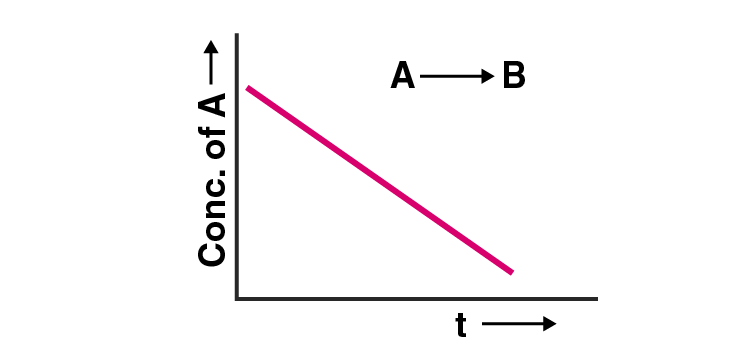Solution:

(i) It is a zero-order reaction as the graph is satisfying the equation [A] = [A0] – kt.

(ii) The slope of the curve is the negative of the rate constant that is denoted by –k.

(iii) Unit of the rate constant is Ms-1 or mol L-1s-1.

43. The reaction between H2(g) and O2(g) is highly feasible yet allowing the gases to stand at room temperature in the same vessel does not lead to the formation of water. Explain.

Solution:

According to Maxwell Boltzmann energy distribution curve when temperature T becomes T+10 degree Celsius then the effective collision and energy of molecules increases resulting in the formation of a product.

44. Why does the rate of a reaction increase with a rise in temperature?

Solution:

Rate of reaction increases with the rise in temperature because at the higher temperature the fraction of particles collision to cross the energy barrier will be large. Therefore the rate also becomes faster.

45. Oxygen is available in plenty in the air yet fuels do not burn by themselves at room temperature. Explain.

Solution:

Oxygen is available in plenty of air yet fuels do not burn by themselves at room temperature because reactants must have a minimum amount of energy known as the activation energy to form a product.

46. Why is the probability of reaction with molecularity higher than three very rare?

Solution:

For a reaction having more than three molecules, the proper orientation is not possible that makes these reactions rare. Therefore the probability of more than three molecules colliding simultaneously will be small.

47. Why does the rate of any reaction generally decrease during the reaction?

Solution:

The rate of a reaction decreases because the reactants will proceed to convert into the products. The rate of a reaction always depends on the concentration of the reactants.

48. Thermodynamic feasibility of the reaction alone cannot decide the rate of the reaction. Explain with the help of one example.

Solution:

Thermodynamic feasibility of the reaction alone cannot decide the rate of the reaction because for a reaction to occur it is important for the molecules to have an energy greater than the activation energy.

For example,

Diamond → Graphite ΔG= -ve

ΔG negative means that the reaction is feasible but this reaction is a slow process as energy is less than the activation energy.

49. Why in the redox titration of KMnO4vs oxalic acid, we heat oxalic acid solution before starting the titration?

Solution:

We heat oxalic acid solution because without heating it is a slow process as energy greater than the activation energy is required for a reaction. So to increase the energy, the temperature must be increased which is only possible by heating the oxalic acid solution.

50. Why can’t molecularity of any reaction be equal to zero?

Solution:

Zero molecularity means there is no reactant, as a result of which a reaction cannot occur. So if there is a reaction then its molecularity will be greater than zero.

51. Why is molecularity applicable only to elementary reactions and order is applicable for elementary as well as complex reactions?

Solution:

Molecularity is applicable only for elementary reactions as they are the single-step reactions and the rate depends on the concentration of each molecule, whereas in case of complex reactions there are multiple reactions involved and thus molecularity holds no meaning.

52. Why can we not determine the order of a reaction by taking into consideration the balanced chemical equation?

Solution:

Order is an experimental quantity. For example, in complex reactions, the rate and order are dependent on the slow step reactions, so in such cases, the order is not completely dependent on the balanced chemical equations. We cannot determine the order by taking into consideration the balanced chemical equation.

### Class 12 Chemistry NCERT Exemplar Problems on Chemical Kinetics

Chemical kinetics is the study and discussion of chemical reactions with respect to reaction rates, the effect of various variables, the formation of intermediates and the re-arrangement of atoms. Solve the NCERT Exemplar Class 12 Chemistry Chapter 4 Chemical Kinetics for a better understanding of the chapter. Chemical kinetics is a fundamental topic which is important for the Class 12 board examination.

#### Key points in this exemplar solution are as follows:

1. The Rate of a Chemical Reaction – Formula Derivation and Numerical Problems
2. Factors Influencing the Rate of a Reaction
1. Dependence of Rate on Concentration
2. Rate Expression and Rate Constant
3. Order of a Reaction
4. Molecularity of a Reaction
3. Integrated Rate Equations
1. Zero Order Reactions
2. First-Order Reactions
3. Half-Life of a Reaction
4. Pseudo First Order Reaction
5. Temperature Dependence of the Rate of a Reaction
1. Effect of Catalyst Ex 4.6 – Collision Theory of Chemical Reactions

### Why Opt for BYJU’S

BYJU’S provides NCERT Class 12 notes, study materials, NCERT solutions, exemplar questions, sample papers and previous years’ question papers. To access and download all NCERT Class 12 resources, download BYJU’S – The Learning App or visit BYJU’S website.